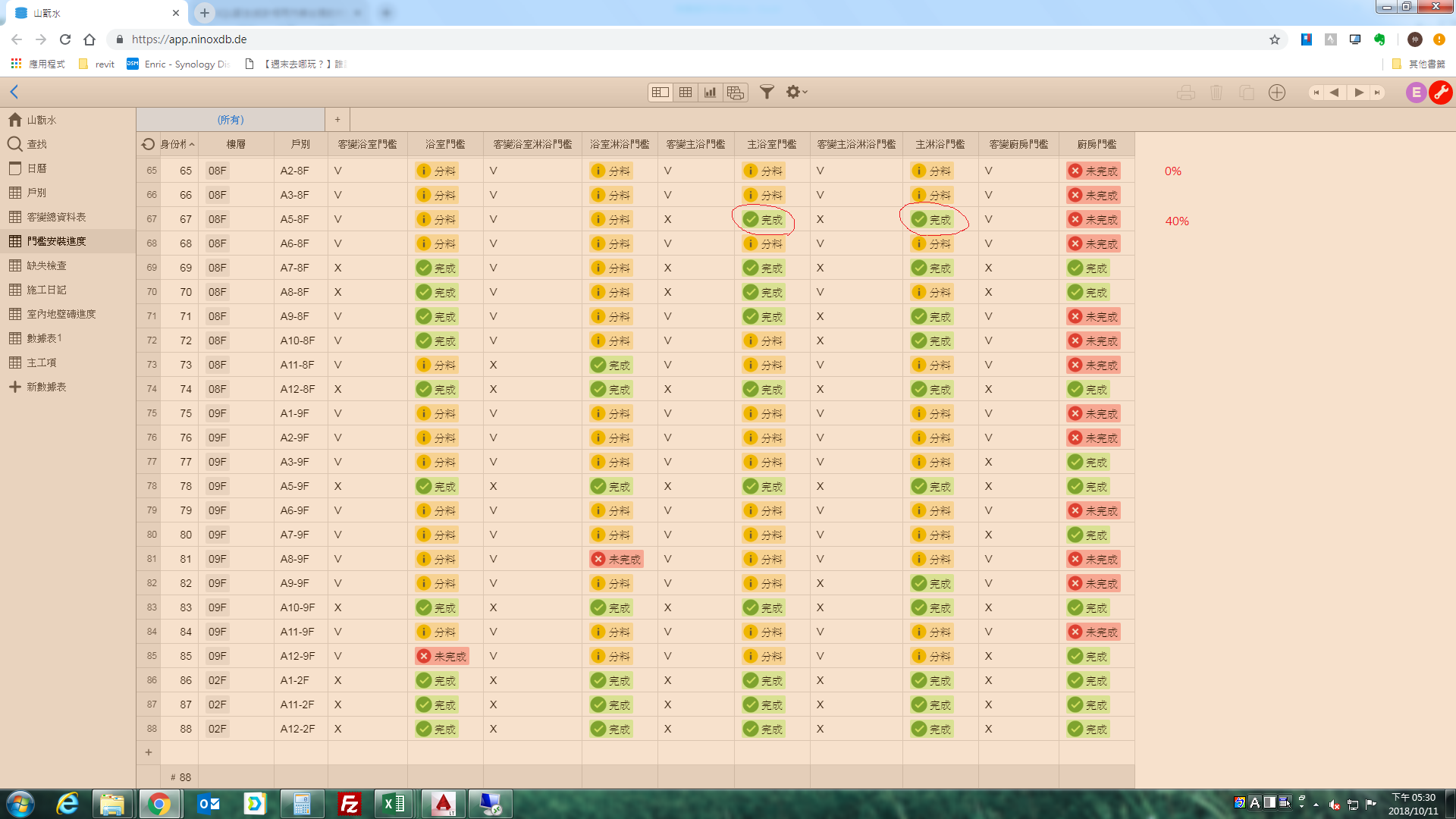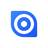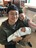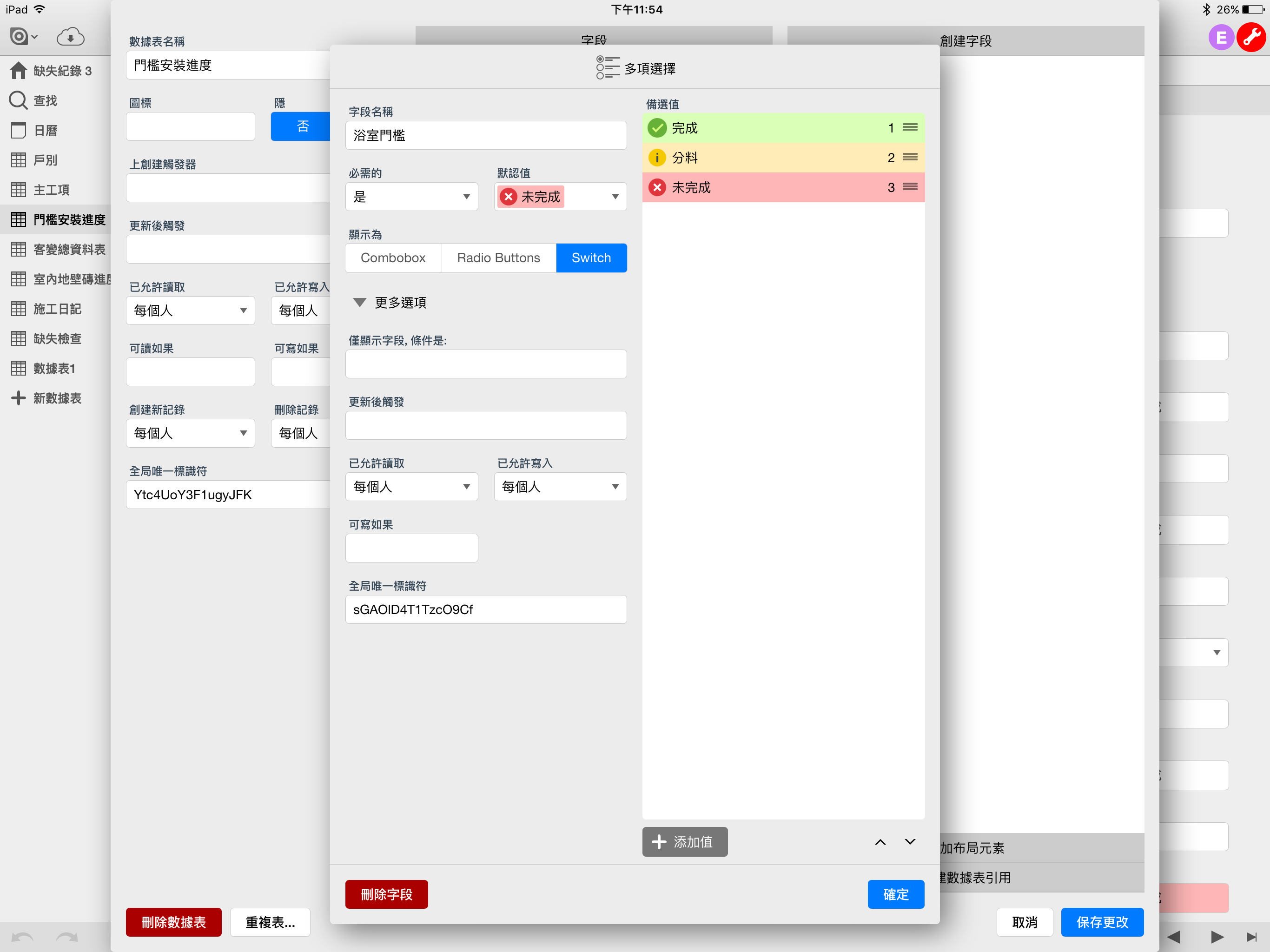How to count non-digital content and calculate the percentageI want to count the completed quantity and calculate the percentage of completion. How can I do it? thank you.Hi,

you can use a formula like this for it:

let myfield1 := if text(Field1) = "Yes" then 20 end;
let myfield2 := if text(Field2) = "Yes" then 20 end;
myfield1 + myfield2

You just need to adjust the formula to your field names and the amount your field (as I assume you have five, thats why I chose the 20 for each variable...).

Best, JörgWhich position should the formula be filled in? Thanks.Which position should the formula be filled in? Thanks.Hi,

please put the formula in a formula field.

Best, Jörg

Thank you, Jörg